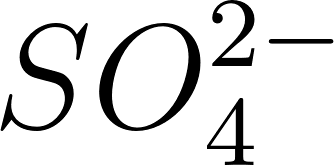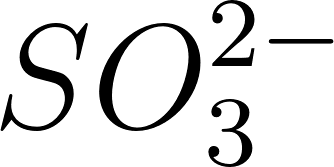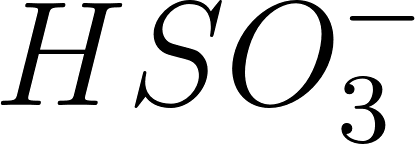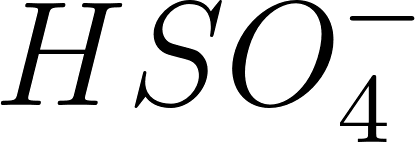×
Get Full Access to Introductory Chemistry - 5 Edition - Chapter 16 - Problem 57p
Get Full Access to Introductory Chemistry - 5 Edition - Chapter 16 - Problem 57p

×

# Assign an oxidation state to each element in each reaction and use the change inISBN: 9780321910295 34

## Solution for problem 57P Chapter 16

Introductory Chemistry | 5th Edition

• Textbook Solutions
• 2901 Step-by-step solutions solved by professors and subject experts
• Get 24/7 help from StudySoup virtual teaching assistantsIntroductory Chemistry | 5th Edition

4 5 1 302 Reviews
14
4
Problem 57P

What is the oxidation state of S in each ion?

(a)(b)(c)(d)Step-by-Step Solution:

Step 1 of 5

The following rules should be followed to calculate the oxidation number of an element in the compound.

1) The oxidation number of the atoms in any free, uncombined element, is zero

2) The sum of the oxidation numbers of all atoms in a compound is zero

3) The sum of the oxidation numbers of all atoms in an ion is equal to the charge of the ion

4) The oxidation number of fluorine in all its compounds is5) The oxidation number of other halogens in their compounds is usuallyStep 2 of 5

Step 3 of 5

##### ISBN: 9780321910295

Introductory Chemistry was written by and is associated to the ISBN: 9780321910295. Since the solution to 57P from 16 chapter was answered, more than 1062 students have viewed the full step-by-step answer. The answer to “?What is the oxidation state of S in each ion?(a) (b) (c) (d)” is broken down into a number of easy to follow steps, and 13 words. This full solution covers the following key subjects: element, state, Being, sbcl, oxidation. This expansive textbook survival guide covers 19 chapters, and 2046 solutions. The full step-by-step solution to problem: 57P from chapter: 16 was answered by , our top Chemistry solution expert on 05/06/17, 06:45PM. This textbook survival guide was created for the textbook: Introductory Chemistry, edition: 5.

## Discover and learn what students are asking

Calculus: Early Transcendental Functions : Change of Variables: Polar Coordinates
?Describing a Region In Exercises 5-8, use polar coordinates to describe the region shown.

Statistics: Informed Decisions Using Data : Inference about the Difference between Two Medians: Dependent Samples
?In Problems 3–10, use the Wilcoxon matched-pairs signedranks test to test the given hypotheses at the a = 0.05 level of significance. The dependent sa

Unlock Textbook Solution